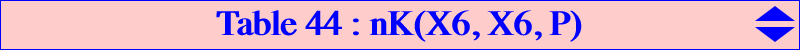The Euler pencil of circles is the pencil generated by the circumcircle (O) and the nine point circle (N) of ABC. It contains several other well-known circles namely the polar circle (P), the orthoptic circle (S) of the Steiner in-ellipse, the tangential circle (T), the orthocentroidal circle (OC) and the decomposed circle (L) which is the union of the line at infinity and the radical axis of the pencil. This is the orthic axis of ABC i.e. the trilinear polar of the orthocenter H. Some other circles are mentioned below. Each circle (C) has an equation of the form : (2 - t) (SA x^2 + SB y^2 + SC z^2) - 2 (a^2 y z + b^2 z x + c^2 x y) = 0, or more loosely : (C) = (2 - t) (P) - 2 (O). The center X of (C) is the point of the Euler line such that : HX = 2 / (4 - t) HO (vectors). *** The locus of point M such that the pedal circle of M (and its isogonal conjugate M*) is orthogonal to (C) is the cubic (K) with equation : t S^2 x y z + ∑ a^2 x (c^2 y^2 + b^2 z^2) = 0 with S = 2*area(ABC) hence (K) is an isogonal non-pivotal cubic with root the Lemoine point K = X(6). All these cubics form a pencil generated by the union of the sidelines of ABC (when (C) is the polar circle) and Kjp = K024 (when (C) is the nine point circle). They all contain A, B, C, the centers Ωa, Ωb, Ωc of the 3 Apollonian circles and they are tangent at A, B, C to the sidelines of the antimedial triangle. Any cubic (K) meet the circumcircle (O) and the line at infinity at the same nine points that also lie on the isogonal nK0 whose root is the point R on the line GK = X(2)X(6) defined by : R = SO X(6)- 8 t S^2 X(2) where SO = (a^2 + b^2 + c^2)/2 as usual. The following table shows a selection of the most remarkable cubics of this pencil (K).t X (C) (K) R remarks ∞ X(4) (P) splitted cubic X(2) (K) is the union of the sidelines of ABC 0 X(5) (N) K024 X(6) (K) is a nK0+ 2 X(3) (O) K191 ? 4 X(30) (L) K192 X(230) (K) is a focal cubic 1 X(2) (S) K634 X(5304) -8 X(3845) see note 1 - 4 R / r see note 2 K635 X(5275) (K) is a nodal cubic (csc w)^2 X(1513) splitted cubic X(385) (K) splits into the Lemoine axis and the Steiner in-ellipse. w is the Brocard anglenote 1 : (C) is the circle of the pencil orthogonal to the Moses circle. note 2 : (C) is the circle of the pencil orthogonal to the incircle.